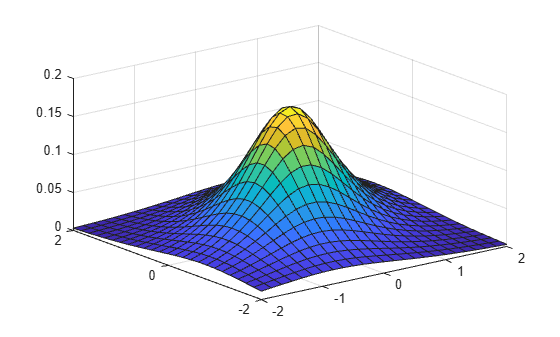# mvtpdf

Multivariate t probability density function

## Syntax

```y = mvtpdf(X,C,df) ```

## Description

`y = mvtpdf(X,C,df)` returns the probability density of the multivariate t distribution with correlation parameters `C` and degrees of freedom `df`, evaluated at each row of `X`. Rows of the n-by-d matrix `X` correspond to observations or points, and columns correspond to variables or coordinates. `C` is a symmetric, positive definite, d-by-d matrix, typically a correlation matrix. If its diagonal elements are not 1, `mvtpdf` scales `C` to correlation form. `mvtcdf` does not rescale `X`. `df` is a scalar, or a vector with n elements. `y` is an n-by-1 vector.

## Examples

collapse all

Compute the pdf of a multivariate t distribution with correlation parameters `C = [1 .4; .4 1]` and 2 degrees of freedom.

```[X1,X2] = meshgrid(linspace(-2,2,25)',linspace(-2,2,25)'); X = [X1(:) X2(:)]; C = [1 .4; .4 1]; df = 2; p = mvtpdf(X,C,df);```

Plot the pdf.

```figure; surf(X1,X2,reshape(p,25,25))```